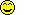# Math Is Fun Forum

Discussion about math, puzzles, games and fun.   Useful symbols: ÷ × ½ √ ∞ ≠ ≤ ≥ ≈ ⇒ ± ∈ Δ θ ∴ ∑ ∫  π  -¹ ² ³ °

You are not logged in.

## #1 2005-11-21 04:57:02

mikau
Member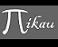Registered: 2005-08-22
Posts: 1,504

### proof of the sum of squares

I was reading proofs for the exact area under a curve, (as the number of rectangles increases without bound) and right out of no where they replaced a sum of n squared integers into a differant expression and simply said "since 1^2 + 2^2 + 3^2 +... + n^2 = n(n + 1)(2n + 1)/6." Uh... it does? I'm certain they never told me that before. I'd remember something that cool. I thought maybe they'd explain it later when I learn about definite integrals, but they just mentioned it as if I already knew it. Never heard it before.

Does anyone know the proof for this formula? Or do I need to know more about calculus before I can understand it?

Last edited by mikau (2005-11-21 04:57:58)

A logarithm is just a misspelled algorithm.

Offline

## #2 2005-11-21 10:43:28

ryos
MemberRegistered: 2005-08-04
Posts: 394

### Re: proof of the sum of squares

I didn't learn that one till college algebra...

El que pega primero pega dos veces.

Offline

## #3 2005-11-21 13:07:04

mikau
MemberRegistered: 2005-08-22
Posts: 1,504

### Re: proof of the sum of squares

Induction? I'd like a proof. It appears my mathbook assumes I know it. Must have been an updated version of a previous book I didn't know about.

Last edited by mikau (2005-11-21 13:07:21)

A logarithm is just a misspelled algorithm.

Offline

## #4 2005-11-22 05:05:57

mathsyperson
Moderator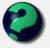Registered: 2005-06-22
Posts: 4,900

### Re: proof of the sum of squares

I found a proof in my maths book. I tried scanning and uploading it, but that didn't seem to work.
Anyway, here it is:

Consider the identity 24r² + 2 (2r+1)³ - (2r-1)³
and take r = 1, 2, 3, ..., n.

24(1²) + 2 = 3³ - 1³
24(2²) + 2 = 5³ - 3³
24(3²) + 2 = 7³ - 5³
....................................
24[(n-1)²] + 2 = (2n-1)³ - (2n-3)³
24[(n)²] + 2 = (2n+1)³ - (2n-1)³

24[1²+2²+3²+...+(n-1)²+n²] + 2n = (2n+1)³ - 1³

That is: 24 [sum of all squares up to n²] + 2n = 8n³ + 12n² + 6n

24[sum of all squares up to n²] = 8n³ + 12n² + 4n
= 4n(2n² + 3n + 1)
[sum of all squares up to n²] = (n/6)(2n² + 3n + 1)
= (n/6)(2n+1)(n+1)

Why did the vector cross the road?
It wanted to be normal.

Offline

## #5 2005-11-22 09:54:14

mathsyperson
ModeratorRegistered: 2005-06-22
Posts: 4,900

### Re: proof of the sum of squares

kylekatarn wrote:

BTW, mathsy, its much more easier if you work with induction and ∑-forms.

True, but I think that my book's proof is quite elegant, with all the cancelling out on the right-hand side. Plus, I'd had a hard day at school, so I didn't want to think.

kylekatarn also wrote:

Induction? I'd like a proof.

Mathematical Induction provides proofs...

Also true. The proof by induction would go something like this:

First, prove that ((n(n+1)(2n+1))/6 = 0² when n = 0.
((0(0+1)(2*0+1))/6 = 0²

So far, so good. Now we need to prove that the difference when you increase n by 1 is identical in both cases.

The difference when increasing from k to k+1 on the right-hand side is obviously (k+1)².

The left-hand side is slightly trickier.
When the value is k, it would be ((k(k+1)(2k+1))/6 = (2k³ + 3k² + k)/6
When the value is (k+1), it would be (([k+1]([k+1]+1)(2[k+1]+1))/6 = ((k+1)(k+2)(2k+3))/6 = (2k³ + 9k² + 13k + 6)/6

The difference of these is (6k² + 12k + 6)/6, or k² + 2k + 1.

ZOMG PERFECT SQUARE LOLZ.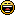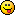k² + 2k + 1 = (k+1)², which is the same result that we wanted from above.

Finally, kylekatarn wrote:

Less words, more math.
[2b | ~2b]?

Well, he did...

Why did the vector cross the road?
It wanted to be normal.

Offline

## #6 2008-04-30 23:17:52

_________
Guest

### Re: proof of the sum of squares

I am looking for the proof that any polynomial with complex coefficients can be written as a sum of squares.

## #7 2008-05-01 03:19:25

Member
Registered: 2008-01-16
Posts: 147

### Re: proof of the sum of squares

As I said before ,

Offline

## #8 2009-08-13 10:26:56

lashko
Member
Registered: 2009-08-13
Posts: 8

### Re: proof of the sum of squares

James wrote:

This is the text i want to quote.

Offline

## #9 2009-08-13 10:29:02

lashko
Member
Registered: 2009-08-13
Posts: 8

### Re: proof of the sum of squares

24[(n-1)²] + 2 = (2n-1)³ - (2n-3)³
24[(n)²] + 2 = (2n+1)³ - (2n-1)³

how did u get those equalities?
Thanks

Offline

## #10 2009-08-13 10:46:31

Ricky
Moderator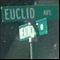Registered: 2005-12-04
Posts: 3,791

### Re: proof of the sum of squares

Lashko, just use the fact that:

"In the real world, this would be a problem.  But in mathematics, we can just define a place where this problem doesn't exist.  So we'll go ahead and do that now..."

Offline

## #11 2009-08-13 14:31:47

i love my horse
Member
Registered: 2009-08-13
Posts: 1

I love math

Offline

## #12 2009-08-14 02:19:29

quittyqat
Member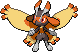Registered: 2009-04-08
Posts: 1,215

### Re: proof of the sum of squares

Hi, i love my horse!
P.S. Click the smiley and it will take you to a screen where it will wave.

Last edited by quittyqat (2009-08-14 02:22:06)

I'll be here at least once every decade.

Offline

## #13 2009-09-29 12:36:58

How if i^3 = [1/2 n (n+1)]^2 ???